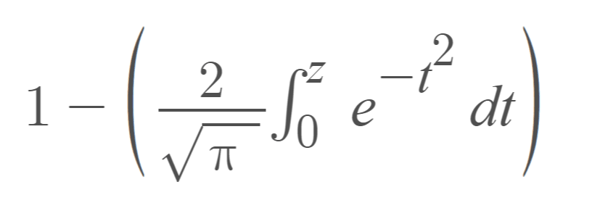Trusted answers to developer questions
Trusted Answers to Developer Questions

Related Tags

golang

# What is the Erfc function in golang?

Faraz Karim

Grokking Modern System Design Interview for Engineers & Managers

Ace your System Design Interview and take your career to the next level. Learn to handle the design of applications like Netflix, Quora, Facebook, Uber, and many more in a 45-min interview. Learn the RESHADED framework for architecting web-scale applications by determining requirements, constraints, and assumptions before diving into a step-by-step design process.

## The Erfc function

The Go programming language uses the Erfc function to find the complementary error function of a certain decimal number.

To use this function, you must import the math package in your file and access the Erfc function within it using the . notation (math.Erfc). Here, Erfc is the actual function, while math is the Go package that stores the definition of this function.

## The complementary error function

The complementary error function is a sigmoid function used in probability, statistics, and partial differential equations. It equals the error function subtracted from 1 (1 - erf(x)). It is represented using erfc(x), and the following formula defines it:## Function definition

The definition of the Erfc function inside the math package is:

## Parameters

Erfc function takes a single argument of type float64. This argument represents the decimal number whose complementary error function you want to find.

## Return value

The Erfc function returns a single value of type float64. This value represents the complementary error function for a particular input argument.

Following are two types of return values only used by the function under certain circumstances:

• NAN: Not a number or NAN is returned in all cases where the input argument is of undefined value.

• 0: The Erfc function returns 0 if the input argument has a value equalling positive infinity.

• 2: The Erfc function returns 2 if the input argument has a value equalling negative infinity.

Giving an empty argument or an argument that is not a numeric value results in an error.

## Examples

Following is a simple example where we find out the complementary error function of a positive decimal value:

package mainimport ("fmt"		"math")func main() {	x := 0.8	y := math.Erfc(x)	fmt.Print("The complementary error function of ", x, " is ", y)}

The following example shows how the Erfc function deals with arguments of infinite value:

package mainimport ("fmt"		"math")func main() {	zero := 0.0	// -1/zeoro is a negatively infinite value	y := math.Erfc(-1/zero)	fmt.Print("The complementary error function of -Inf is ", y)	fmt.Print("\n")	// 1/zeoro is a positively infinite value	x := math.Erfc(1/zero)	fmt.Print("The complementary error function of +Inf is ", x)}

RELATED TAGS

golang

CONTRIBUTOR

Faraz Karim